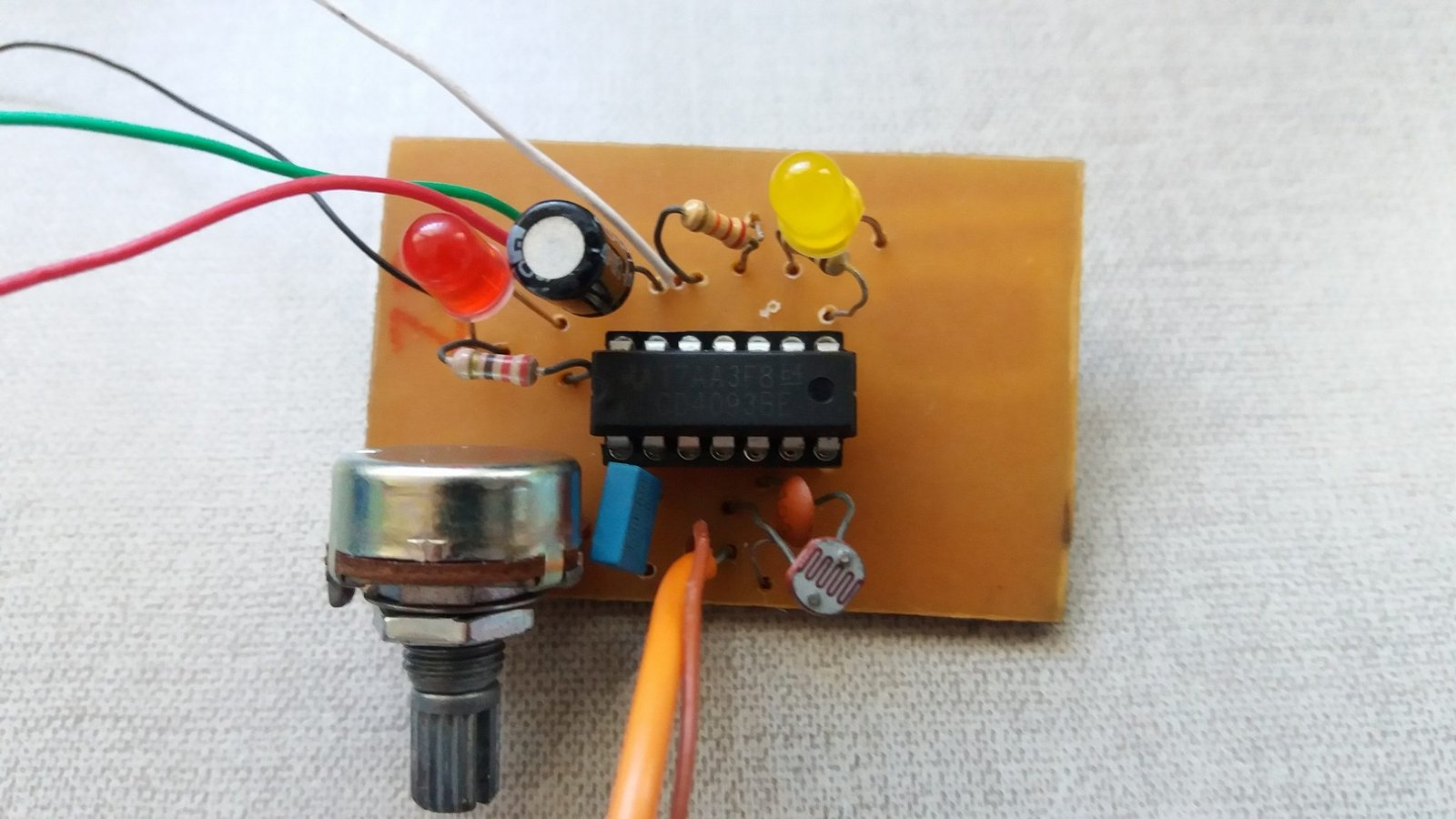# Meet the 4093

This post’s subject is the 4093, it is an integrated circuit very simple and useful to build many electronic projects.

#### How it works?

The 4093 is a CMOS, a family of integrated circuits which have field effect transistors with a silicon oxide layer (in grey) and another with polysilicon (in white) in the transistor’s gate (G). Below are field effect transistors MOSFETs in cross-section.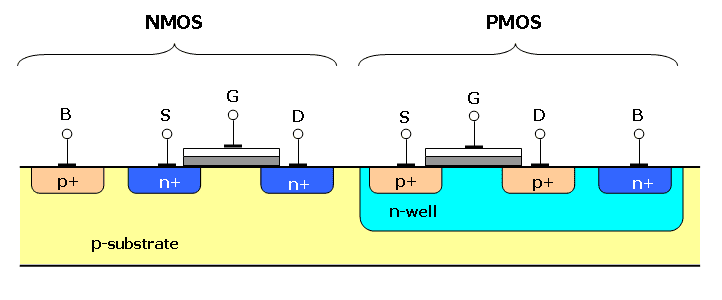Inside 4093, there are 4 NAND logic gates with Schmitt Trigger, can be supplied with voltages from 3 to 15 V. Reminding that VSS is the ground or GND.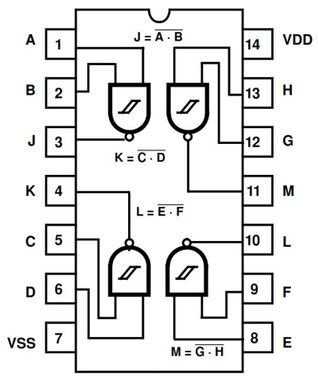To see how the logic gate NAND works, click in the button below, will go to a post about combinational circuits.

What is Schmitt Trigger? It is a comparator circuit which serves to eliminate noises. When the input is above a determined value T, the output will be level high M or “1”, when stays below a certain value -T, the output will be low level -M or “0”.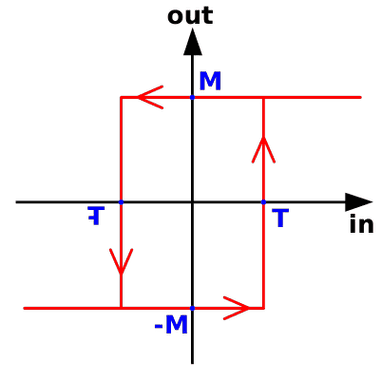These are the Schmitt Trigger’s symbols, the above is the inverter and below is non-inverter.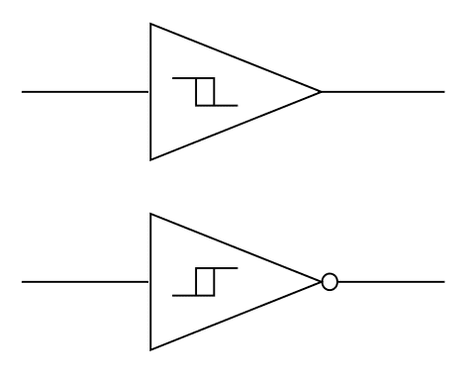This is the Schmitt Trigger’s circuit inside a chip, the input is linked to the NAND gate’s output. The Ps are the PMOS transistors and the Ns are NMOS.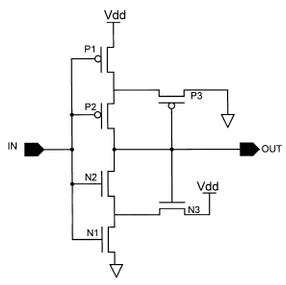#### Some projects

It is possible to build many circuit projects with this chip. This post only show few examples.

• Square wave generator: This circuit produce a square wave. Can put a resistor instead a potentiometer, which serves to control a frequency band. Can change the values of capacitor and potentiometer, or resistor, to get a different frequency.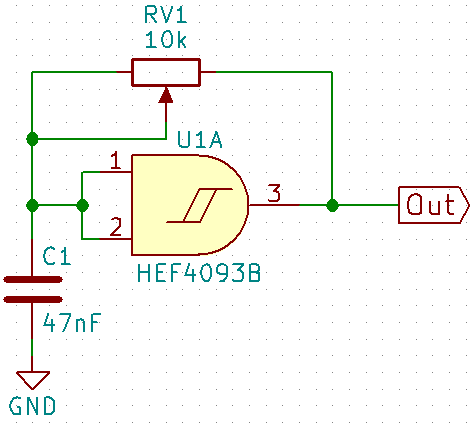• Light sensor: Just replace the resistor with a LDR and there is an oscillator whose frequency is controlled by light intensity.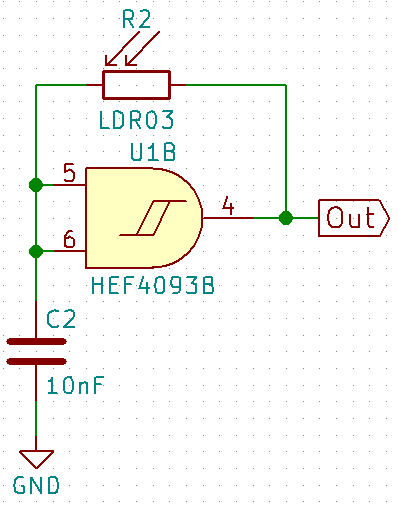This is the frequency equation of this oscillator type.

$f=\frac{1}{RCln(\frac{V_{T}^{+}(V_{S}-V_{T}^{-})}{V_{T}^{-}(V_{S}-V_{T}^{+})})}$

$V_{S}$ is the output voltage value, in this case has the same value of supply voltage $V_{CC}$$V_{T}^{+}$ is the positive limit which generates the high level in output and $V_{T}^{-}$ is the negative limit which produces low level. The difference between $V_{T}^{+}$ and $V_{T}^{-}$ is the hysteresis voltage $V_{H}$. In 4093’s datasheet, these values are shown and depends on the temperature and voltage supply.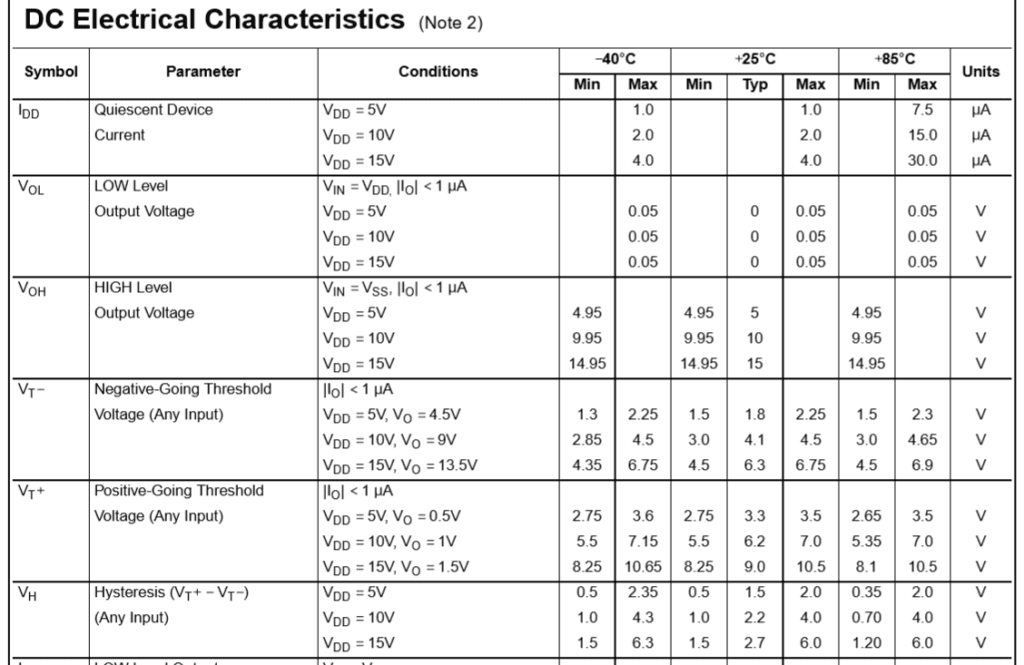• Touch sensor: When touch in the sensor, can be a metallic part or a bare wire, a LED is turned on.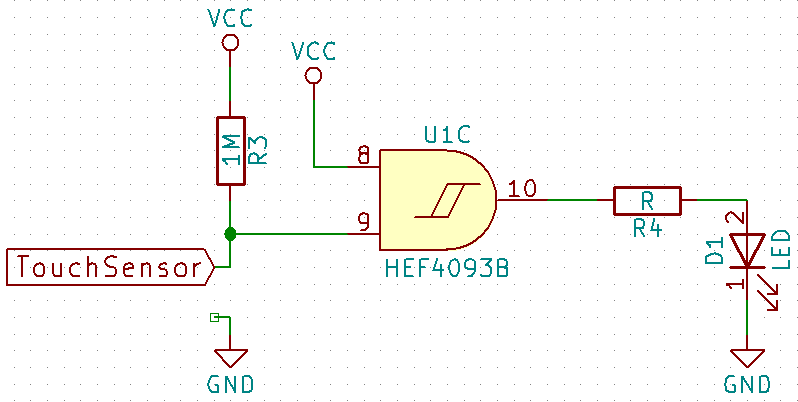• Monostable: When the switch SW1A is closed, the LED is turned on. When it is opened again, the LED stays turned on for a while. When higher the C3 and R5 values, longer will be the time the LED is turned on.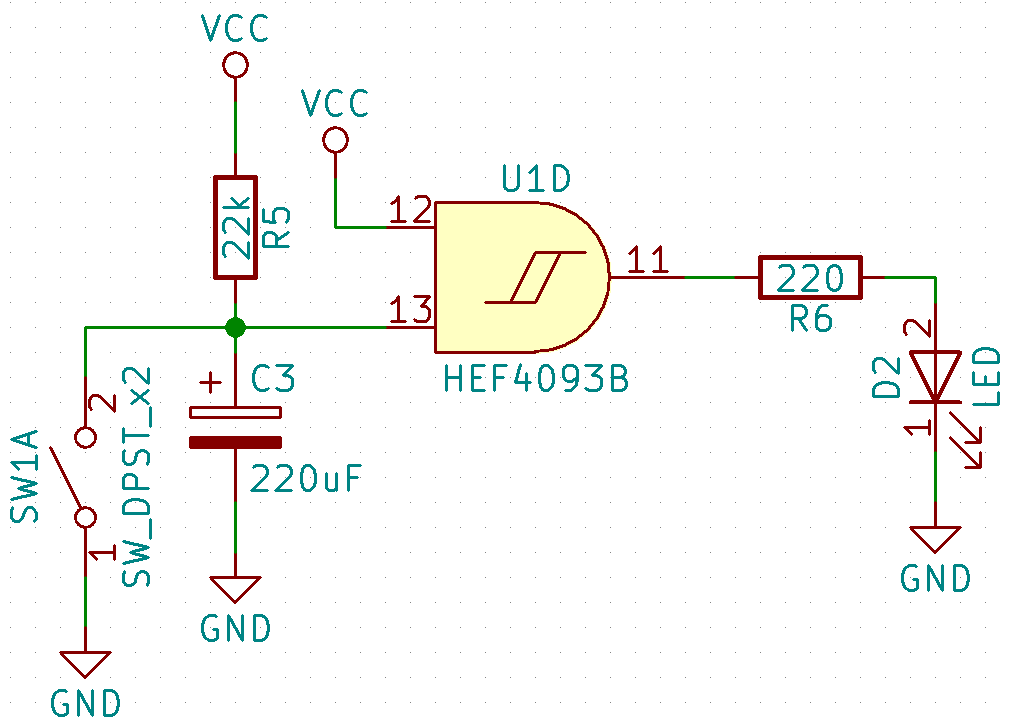The circuits shown above can be assembled in a single printed circuit board.